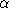# 2D Alpha ShapesReference Manual

Tran Kai Frank Da

This chapter presents a framework for alpha shapes. The description is based on the articles [EM94, Ede92]. Alpha shapes are the generalization of the convex hull of a point set. Let S be a finite set of points ind, d = 2,3 anda parameter with 0. For=, the-shape is the convex hull of S. Asdecreases, the-shape shrinks and develops cavities, as soon as a sphere of radius sqrt() can be put inside. Finally, for= 0, the-shape is the set S itself.

We distinguish two versions of alpha shapes, one is based on the Delaunay triangulation and the other on its generalization, the regular triangulation, replacing the natural distance by the power to weighted points. The metric used determines an underlying triangulation of the alpha shape and thus, the version computed. The basic alpha shape (cf. 29.4.1) is associated with the Delaunay triangulation (cf. 25.5). The weighted alpha shape (cf. 29.4.3) is associated with the regular triangulation (cf. 25.6).

There is a close connection between alpha shapes and the underlying triangulations. More precisely, the-complex of S is a subcomplex of this triangulation of S, containing the-exposed k-simplices, 0kd. A simplex is-exposed, if there is an open disk (resp. ball) of radius sqrt() through the vertices of the simplex that does not contain any other point of S, for the metric used in the computation of the underlying triangulation. The corresponding-shape is defined as the underlying interior space of the-complex.

In general, an-complex is a non-connected and non-pure polytope, it means, that one k-simplex, 0kd-1 is not necessary adjacent to a (k+1)-simplex.

The-shapes of S form a discrete family, even though they are defined for all real numberswith 0. Thus, we can represent the entire family of-shapes of S by the underlying triangulation of S. In this representation each k-simplex of the underlying triangulation is associated with an interval that specifies for which values ofthe k-simplex belongs to the-shape. Relying on this result, the family of-shapes can be computed efficiently and relatively easily. Furthermore, we can select an appropriate-shape from a finite number of different-shapes and corresponding-values.

## 29.5   Classified Reference Pages

### Classes

CGAL::Alpha_shape_2<Dt>
CGAL::Weighted_alpha_shape_euclidean_traits_2<K>
CGAL::Alpha_shape_vertex_base_2<AlphaShapeTraits_2>
CGAL::Alpha_shape_face_base_2<AlphaShapeTraits_2, TriangulationFaceBase_2>